# SUMPRODUCT vs SUMIFS Function in Excel

The SUMPRODUCT function was always the go-to function for finding conditional sums, till the time SUMIFS was introduced in Excel 2007.

A lot of people shifted to using SUMIFS instead of SUMPRODUCT since then, but a good number of people stuck to their guns and continued using the SUMPRODUCT function.

The reason for this is not just familiarity. There are other inherent factors too.

Both functions are available now for Excel users, but many find it tough to decide which one is the better option and which situations each of them are more suitable for.

In this article, we will first take a look at what each of the two functions was meant to do as well as what they are capable of doing.

We will then take a look at how they are similar and how they are different. Finally, we will see the best situations to apply each of the two.

## Why was SUMIFS introduced?

Originally, the SUMIF (without the ‘s’) function was used for simple conditional sums. However, this function had a major drawback.

It could only be used to find the sum based on a single condition (or criterion).

Interestingly, some Excel users found a way around this. They used the SUMPRODUCT function (which was originally meant to find the sum of products of column values) to perform the same task as the SUMIF function, but with more than one criteria.

Eventually, Microsoft realized the necessity of a SUMIF function that could work on more than one criterion. So, they released the new and improved SUMIFS function.

With this function, Excel users can now find sums based on any number of criteria.

## What does SUMIFS function do?

The SUMIFS function finds the sum of values based on one or more criteria.

Its general syntax is:

`=SUMIFS(sum_range, criteria_range, criteria,[ criteria_range2, criteria2, ... criteria_range_n, criteria_])`

To better understand this, let’s take an example. In the table below, there are 10 students with their classes, date of admission and Exam scores. The above function can work with n number of criteria.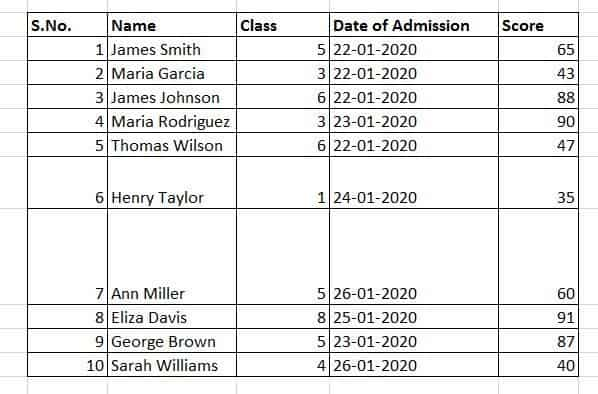To find the total score of students of Class 6, we can write the following in the formula bar:

`=SUMIFS(F3:F12,D3:D12,"=6")`

Here,

• The sum_range includes the cells in locations F3:F12  – The Student Scores
• The criteria_range is in cell locations D3:D12 – The Class of the student
• The criteria is “=6”. This means ‘the Class of the student should be equal to 6’

Here’s what you get as the result: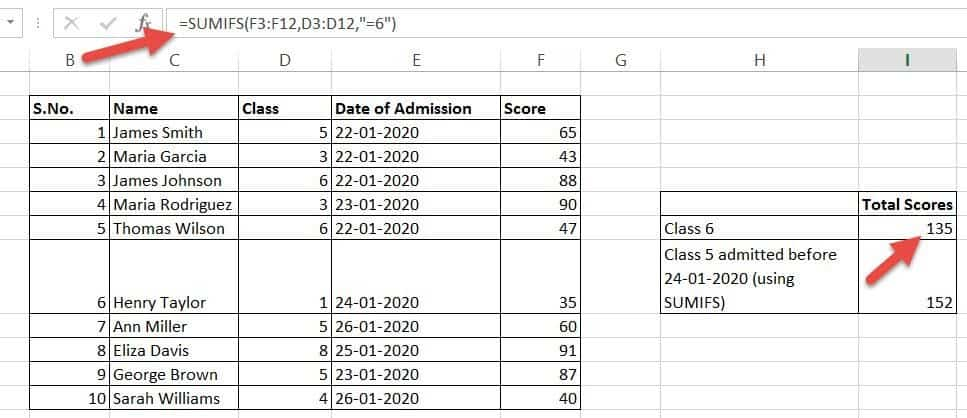You can also find the sum based on more criteria. For example, if you want to find the total score of students only the students of class 5 who were admitted before 24-01-2020 then you can use the same formula as follows:

`=SUMIFS(F3:F12,D3:D12,"=5",E3:E12,"<24-01-2020")`

Here,

• The sum_range includes the cells in locations F3:F12  – The Student Scores
• The first criteria_range is in cell locations D3:D12 – The Class of the student
• The first criteria is “=5”. This means ‘the Class of the student should be equal to 5’
• The second criteria_range is in cell locations E3:E12– The admission date of the student
• The second criteria is “<24-01-2020”. This means ‘the admission date of the student should be less than 24-01-2020’.

Here’s what you get as the result: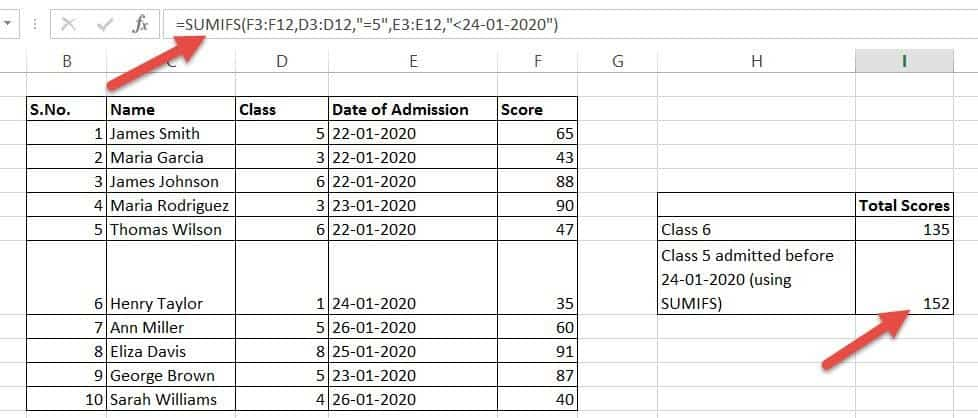## What does the SUMPRODUCT function do?

The SUMPRODUCT function, as mentioned above, was initially created to find the sum of products. This is how it was meant to be used: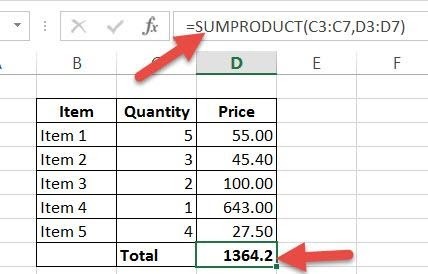Here, all the function does is take each Quantity value (Range C3:C7), multiply it with each Price value (Range D3:D7) and then find the sum of each of these individual products. So it does the following calculation in the background:

`=(C3* D3)+(C4*D4) +(C5*D5) +(C6*D6) +(C7*D7)`

However, Excel users found that this function is much more versatile than that. The SUMPRODUCT function can also be used for a whole lot more than just finding the sum of products. Some of its other applications include:

• Finding the sum of cell values based on multiple conditions
• Finding the count of values that satisfy one or more conditions
• Finding the sum of cell values based on ‘or’ conditions
• Finding the sum of cell values based on ‘and’ conditions

## Using SUMPRODUCT instead of SUMIFS

SUMPRODUCT is capable of performing the same action as the SUMIFS function. To use SUMPRODUCT for finding the sum of values based on one or more criteria, this is the syntax that you can use:

`=SUMPRODUCT((sum_range)*((condition1)*(condition2)*....(condition_n)))`

Here,To understand this better let’s take the same example of student classes, admission dates, and scores. To find the total score of only the students of class 5 who were admitted before 24-01-2020, we can write the following in the formula bar:

• The sum_range includes the cells in locations F3:F12  – The Student Scores
• The first condition is D3:D12=5. This means the ‘the Class of the student should be equal to 6’
• The second condition is E3:E12<“24-1-2020”. This means ‘the admission date of the student should be less than 24-01-2020’.

Here’s what you get as a result: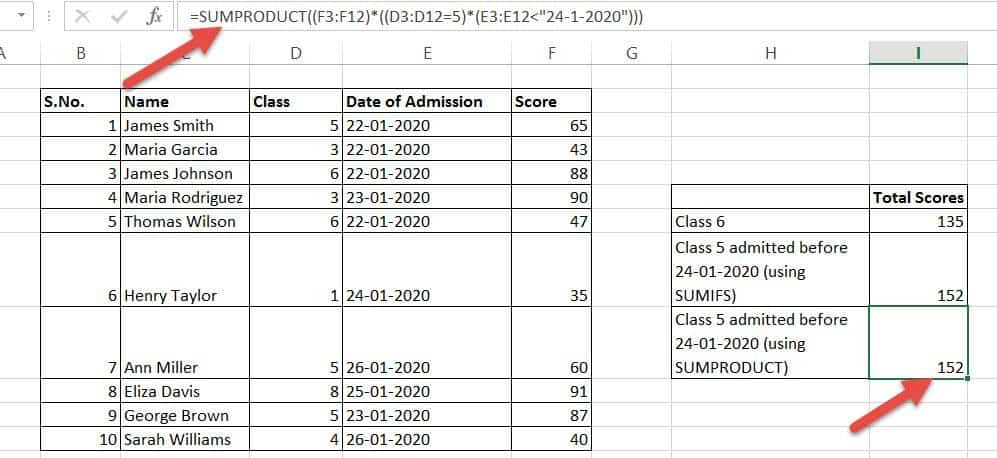Notice you get the same result as when you used the SUMIFS function.

To answer this question we need to break down what just happened. When we use the SUMPRODUCT function in this way, it takes each value of F3:F12 (the score values) and multiplies it with the truth value of each of the given conditions. If the condition is true, SUMPRODUCT multiplies the score with 1 and if it is false, SUMPRODUCT multiplies it with 0.

This will get clearer if you see the image below: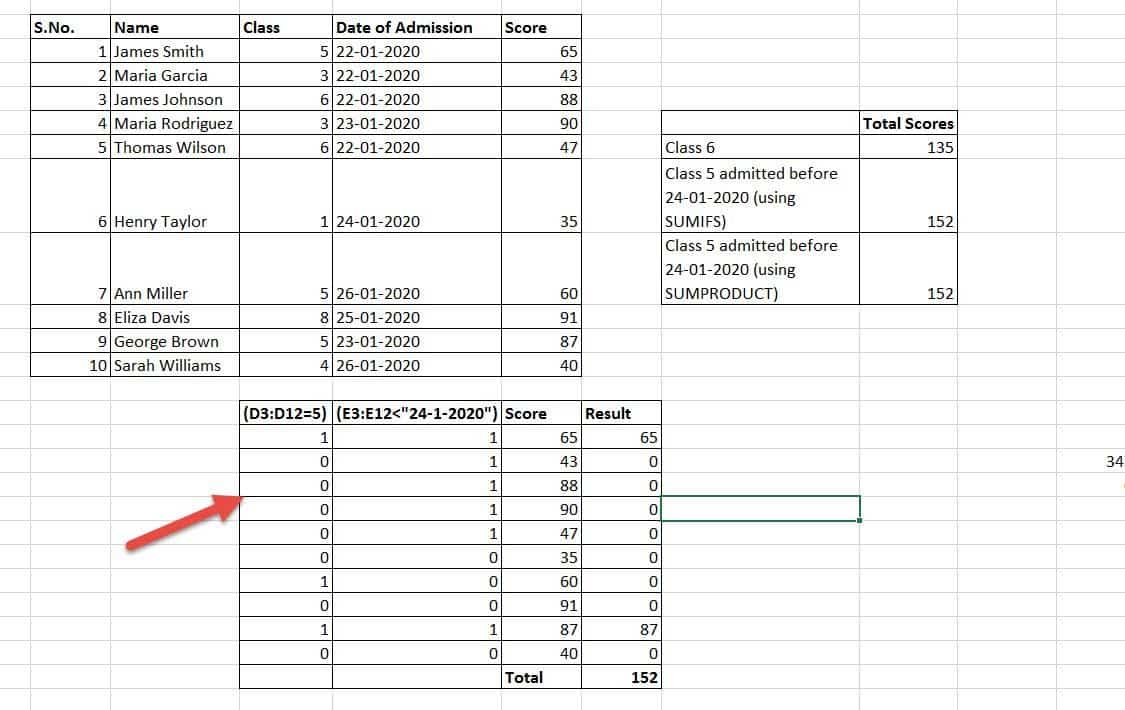If you look at the first row, you will find that condition1 (D3=5) is True , so it is converted to 1. Condition2 (E3<“24-1-2020”) is also True (since the date 22-01-202 is less than 24-01-2020). Therefore it is converted to 1. So for the first row you get the product 1 * 1 * 65, which gives the result 65.

Similarly, in the second row, condition1 is False, so it is converted to 0. Condition2 is True so it is converted to 1. So, for the second row, you get the product 0*1*43, which gives the result 0. That means the second row’s score is ignored.

In this way when you sum up all the products, you get 152 as the final result.

## SUMPRODUCT vs. SUMIFS – How are they Different?

We have highlighted in the table below, the basic differences between SUMPRODUCT and SUMIFS

 SUMPRODUCT SUMIFS SUMPRODUCT is more mathematical calculation-based. SUMIFS is more logic-based. SUMPRODUCT can be used to find the sum of products as well as conditional sums. SUMIFS cannot be used to find the sum of products. SUMPRODUCT is more versatile and flexible. You can do a lot more with it. SUMIFS can only find conditional sums. SUMPRODUCT only works with equal-sized cell ranges. SUMIFS does not require cell ranges to be equal. SUMPRODUCT takes more time to calculate. Using SUMIFS optimizes the speed of calculations. You can check for conditions that involve ‘or’ operations with SUMPRODUCT. For example, if you want to find the scores of all students of class 5 or 6, you can type: =SUMPRODUCT((F3:F12)*((D3:D12=5)+(D3:D12=6))) You cannot use SUMIFS to perform ‘or’ operations.

## How are they Similar?

In spite of the above differences, the two functions do have some degree of similarity. Both functions can be used to find conditional sums and both can work with more than one condition.

This is why some people tend to use them interchangeably, while some get confused between the two. The trick is to know when to use either.

## When Should You Use the SUMIFS Function?

The SUMIFS function is simpler, so if you want to avoid complexity in your calculations, SUMIFS could be a better option for you. Here are three situations in which using SUMIFS is preferred:

• When your sum_range and criteria_range sets are not equal-sized.
• When processing speed is your concern.
• When you feel overwhelmed to see complex formulas with lots of parentheses.

## When Should You Use the SUMPRODUCT Function?

SUMPRODUCT is more universal and versatile in nature, so you can easily apply it to a number of situations. Particularly, here are three situations in which using SUMPRODUCT is preferred:

• When your conditional sum involves an ‘or’ operation
• When you need to find a conditional count, for example, if you want to find how many students are from grade 5. You could also use COUNTIFS for this, though.
• When your conditional sum also involves other calculations, for example, if you want to find the conditional average scores of students.

It is important to remember that SUMPRODUCT functions can slow down processing, so if your sheet involves a large number of conditional sums, it is advisable to avoid using it.